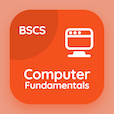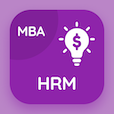Computer Science Online Courses

Computer Basics Quizzes

Computer Basics Quiz PDF - Complete

# Spreadsheet Program Functions Multiple Choice Questions p. 212

Study Spreadsheet Program Functions multiple choice questions and answers, spreadsheet program functions quiz answers PDF 212 to study Computer Basics course online. Spreadsheet Programs MCQ trivia questions, Spreadsheet Program Functions Multiple Choice Questions (MCQ) for online college degrees. "Spreadsheet Program Functions MCQ" PDF eBook: spreadsheet program cells, introduction to internet, input devices of computer, read only memory (rom), spreadsheet program functions test prep for computer software engineer online degree.

"In a computer, third part of number format describes" MCQ PDF: negative number, positive number, zero values, and text values for computer software engineer. Learn spreadsheet programs questions and answers to improve problem solving skills to learn online certificate courses.

MCQ: In a computer, third part of number format describes

positive number
negative number
zero values
text values

MCQ: Which of the following is not a type of computer's memory ROM?

PROM
EPROM
EEPROM
FPROM

MCQ: Inventor of computer QWERTY keyboard is

Henry Mill
Carlos Glidden
Alexander Graham Bell
Christopher Latham Sholes

MCQ: Collection of millions of computer interlinked to one another is called

internet
collection
group

MCQ: Raw numbers used in a computer spreadsheet are called

labels
values
formulas
calculations

### More Quizzes from Computer Basics Course